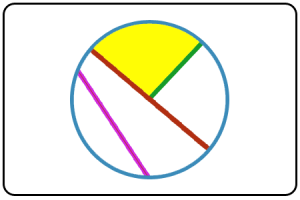Select Page

We’ve been talking geometry in class recently. We’ve discussed polygons (Mrs. Yollis class has a great post about them), angles, and now circles.We began by reviewing all the different parts of a circle. In the picture above, you’ll see a green line segment extending fro the center point of the circle to the edge of a circle. This is called the radius. The red line segment extending from one side of the circle to the other while also going through the center point is our diameter. Much like the radius, the diameter MUST always go through the center of the circle. The diameter is always twice the size of the radius so we can find it by using the formula d=2r. Likewise, because the radius is half the size of the diameter, you can find that by doing r=d ÷ 2.

There are also other parts to a circle as well. The purple line segment is called a chord. The chord must also have two points on the edge of the circle but does not have to go through the center. You also have the yellow shaded in part which is called the sector. The sector is a section of the circle created between to radii and an arc (the arc is a portion of the outside of the circle).The whole outside of the circle is called the circumference. If you were to cut the outside of the circle and lay out out in a straight line, the diameter would fit into the circumference about 3.14 times. This is a number that is referred to as pi. It is often shown using the symbol π. Based upon the relationship between the diameter and the outside of the circle, we can find the circumference by using the formula C = dπ.

Sam, Fiona, Wes, and Ryan from the math movie network set out to make sense of circles and more specifically, circumference. The video below will share with you the meaning of pi as well as how to use it to find the circumference of a circle!

What are some of the different places you find circles in everyday life?

Does circumference make more sense to you now?